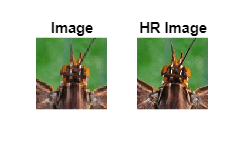# Deploy Super Resolution Application That Uses TensorFlow Lite (TFLite) Model on Host and Raspberry Pi

This example shows simulation and code generation for a super resolution application by using a Tensorflow Lite model. The task of recovering a high resolution (HR) image from its low resolution counterpart is commonly referred to as Single Image Super Resolution (SISR). In this example, you use TFLite to run inference on a pretrained ESRGAN (ESRGAN: Enhanced Super-Resolution Generative Adversarial Networks) model.

The TFLite model used in this example upsamples a 50x50 low resolution image to a 200x200 high resolution image (scale factor=4).

This example is supported for host Windows® and Linux® platforms.

This example uses TFLite to run inference on a pretrained ESRGAN model.

Run these commands to download the ESRGAN network from the URL mentioned below.

```if ~exist("esrgan-tf2.tflite","file") disp('Downloading 5 MB ESRGAN model file...'); url = "https://tfhub.dev/captain-pool/lite-model/esrgan-tf2/1?lite-format=tflite"; websave("esrgan-tf2.tflite",url); end```
```Downloading 5 MB ESRGAN model file... ```

You can use any other super resolution model test this workflow by following the steps mentioned below. If you have only a TensorFlow model for your network, use these steps to convert the TensorFlow model to a TFLite model.

Run these commands to download the image from the URL mentioned below.

```if ~exist("inputsuperresolution","file") disp('Downloading input file...'); url = "https://raw.githubusercontent.com/tensorflow/examples/master/lite/examples/super_resolution/android/app/src/main/assets/lr-1.jpg"; websave("inputsuperresolution.jpg",url); end```
```Downloading input file... ```

Read the input image and display it.

```I = imread('inputsuperresolution.jpg'); imshow(I); title('Original Image');```### The `super_resolution_predict` Entry-Point Function

In this example, you generate code for the entry-point function `super_resolution_predict.m`. This function loads the ESRGAN model into a persistent network object by using the `loadTFLiteModel` function. Then the function performs prediction by passing the network object to the `predict` function. Subsequent calls to this function reuse this persistent object.

`type super_resolution_predict.m`
```function out = super_resolution_predict(in) persistent net; if isempty(net) net = loadTFLiteModel('esrgan-tf2.tflite'); net.Mean = 0; net.StandardDeviation = 1; % Set number of threads based on max number of threads in host / hardware % net.NumThreads = 4; end out = net.predict(in); %% Post processing Output % Cast output to uint8 out = uint8(out); end ```

The `loadTFLiteModel` function creates a `TFLiteModel` object. The properties of this object contain information about the model such as the number and size of inputs and outputs of the model.

```net = loadTFLiteModel('esrgan-tf2.tflite'); % Display Input Size disp(net.InputSize);```
``` {[50 50 3]} ```
```% Display Output Size disp(net.OutputSize); ```
``` {[200 200 3]} ```

### Resize Image Based on TFLite Model Input Shape

Use `imresize` to resize the input image based on TFLite model input shape.

`I = imresize(I,net.InputSize{1}(1:2));`

To perform inference by using the loaded TFLite model, use any of the following workflows.

### Workflow 1: Perform Super Resolution by Using Simulation on Host

Run the simulation by passing the input image `I` to the entry-point function.

`predictionScores = super_resolution_predict(I);`

### Workflow 2: Perform Super Resolution by Running Generated MEX on Host

• MATLAB® Coder™

This example uses the `codegen` command to generate a MEX function that runs on the host platform.

#### Generate MEX Function

To generate a MEX function for a specified entry-point function, create a code configuration object for a MEX. Set the target language to C++.

```cfg = coder.config('mex'); cfg.TargetLang = 'C++';```

Run the `codegen` command to generate the MEX function `super_resolution_predict_mex` on the host platform.

```codegen -config cfg super_resolution_predict -args ones(50,50,3,'uint8') ```

#### Run Generated MEX

Run the generated MEX by passing the input image `I`.

```predictionScores = super_resolution_predict_mex(I); ```

### Workflow 3: Generate Code for Super Resolution Application, Deploy and Run on Raspberry Pi

• MATLAB® Coder™

• Embedded® Coder™

• MATLAB Support Package for Raspberry Pi Hardware. To install this support package, use the Add-On Explorer.

#### Third-Party Prerequisites

• Raspberry Pi hardware

• TFLite library (on the target ARM® hardware)

On the Raspberry Pi hardware, set the environment variable `TFLITE_PATH` to the location of the TFLite library. For more information on how to build the TFLite library and set the environment variables, see Prerequisites for Deep Learning with TensorFlow Lite Models (Deep Learning Toolbox).

#### Set Up Connection with Raspberry Pi

Use the MATLAB Support Package for Raspberry Pi Hardware function `raspi` to create a connection to the Raspberry Pi.

In the following code, replace:

• raspiname with the name of your Raspberry Pi board

```r = raspi('raspiname','username','password'); ```

#### Copy TFLite model to Target Hardware

Copy the TFLite model to the Raspberry Pi board. On the hardware board, set the environment variable `TFLITE_MODEL_PATH` to the location of the TFLite model. For more information on setting environment variables, see Prerequisites for Deep Learning with TensorFlow Lite Models (Deep Learning Toolbox).

In the following commands, replace:

• `targetDir` with the destination folder of TFLite model on the Raspberry Pi board.

```r.putFile('esrgan-tf2.tflite',targetDir) ```

#### Generate PIL MEX Function

To generate a PIL MEX function for a specified entry-point function, create a code configuration object for a static library and set the verification mode to `'PIL'`. Set the target language to C++.

```cfg = coder.config('lib','ecoder',true); cfg.TargetLang = 'C++'; cfg.VerificationMode = 'PIL';```

Create a `coder.hardware` object for Raspberry Pi and attach it to the code generation configuration object.

```hw = coder.hardware('Raspberry Pi'); cfg.Hardware = hw;```

On the host platform, run the `codegen` command to generate a PIL MEX function `super_resolution_predict_pil`.

```codegen -config cfg super_resolution_predict -args ones(50,50,3,'uint8') ```

#### Run Generated PIL MEX

Run the generated PIL executable by passing the input image `I`.

```predictionScores = super_resolution_predict_pil(I); ```

### Display the Super Resolution Image

```subplot(1,2,1); imshow(I); title('Image'); subplot(1,2,2); imshow(predictionScores); title('HR Image');```